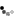Loading... Please wait...

# Convert Cubic Yards

Landscaping mulch, loam and stone is sold by the cubic yard. Cubic yard calculation is made easy when you realize that a cubic yard is 3 feet long on each side (height, width, length). So it contains 27 cubic feet (3x3x3=27).

Which means if it was spread a foot deep, it would cover 27 square feet;

• 6 inches deep =  54 square feet (an area 9 feet by 6 feet)
• 3 inches deep = 108 square feet (an area about 10 feet by 10 feet)
• 2 inches deep = 162 square feet (an area 10 feet by 16 feet).

So if you are covering an area 3 inches deep, divide by the square feet by 108 (or 100 to make it easier)

## Or measure the cubic feet needed and divide by 27:

So measure your area to be covered in feet. Say you have a foundation area to be mulched that is 5 feet by 40 feet. Multiply 5 x 40=200 sq ft.

You want it 3 inches deep. Multiply the 200 sq ft by .25 ft (3 inches is 3/12 or 1/4 of a foot) = 50 cubic feet.

Divide by 27 to get cubic yards - 50 divided by 27 = 1.85 yards. You need two cubic yards.